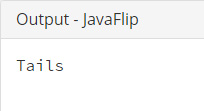# Java Program to Toss a Coin

This Java program is used to toss a coin using Java random class.

• Java Math.random() returns a random value between 0.0 and 1.0 each time.
• If value is below 0.5 then it's Heads or otherwise Tails.
Example:
``````public class JavaFlip {
public static void main(String[] args) {
if (Math.random() < 0.5){
}else{
System.out.println("Tails");
}
}
}
``````
Program Output:Explanation:
In this program, you will learn the code of how the tossing of a coin can be implemented in program. First of all, you have to declare a class name 'JavaFlip' and implement the main() method within this class. Now within this main() method declaration you have to use a conditional statement, in this program, the if() statement within which the Math.random () method, which is a predefined method for randomizing any value is used.

java.lang.Math.random() gives a double value along with the positive sign, greater than or equal to 0.0 and less than 1.0. The resulting values of this predefined method are chosen pseudo-randomly with (approximately) uniform distribution from that range. It does not contains parameter.

if (Math.random()